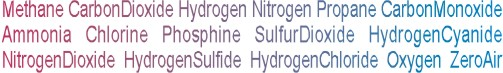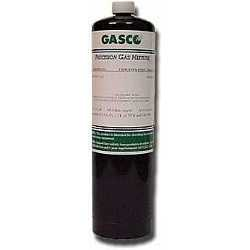Calibration gas blends, mixes and accessories
NON-REACTIVE SINGLE GAS > METHANE :    78 Items found.(1354)

NORLAB Equivalent  P1971075

Norlab Equivalent Calibration Gas 15% LEL (0.75% vol.) Methane Balance air 17L Liter cylinder (Made by Gasco)

Configure

\$36.47(1335)

NORLAB EQUIVALENT  P1971

Norlab Equivalent Calibration Gas 100% Methane Balance - 17L Liter cylinder (Made by Gasco)

Configure

\$36.47(1362)

NORLAB Equivalent  P197125

Norlab Equivalent Calibration Gas 50% LEL (2.5% vol.) Methane Balance air 17L Liter cylinder (Made by Gasco)

Configure

\$36.47(1361)

NORLAB Equivalent  P19712

Norlab Equivalent Calibration Gas 40% LEL (2.0% vol.) Methane Balance air 17L Liter cylinder (Made by Gasco)

Configure

\$36.47(1358)

NORLAB Equivalent  P197115

Norlab Equivalent Calibration Gas 30% LEL (1.5% vol.) Methane Balance air 17L Liter cylinder (Made by Gasco)

Configure

\$36.47(1357)

NORLAB Equivalent  P1971145

Norlab Equivalent Calibration Gas 29% LEL (1.45% vol.) Methane Balance air 17L Liter cylinder (Made by Gasco)

Configure

\$36.47(1355)

NORLAB Equivalent  P19711

Norlab Equivalent Calibration Gas 20% LEL (1.0% vol.) Methane Balance air 17L Liter cylinder (Made by Gasco)

Configure

\$36.47(1353)

NORLAB Equivalent  P1971050

Norlab Equivalent Calibration Gas 10% LEL (0.5% vol.) Methane Balance air 17L Liter cylinder (Made by Gasco)

Configure

\$36.47(1351)

NORLAB Equivalent  P1971050VN

Norlab Equivalent Calibration Gas 50% vol. Methane Balance nitrogen 17L Liter cylinder (Made by Gasco)

Configure

\$36.47(1350)

NORLAB Equivalent  P1971020VN

Norlab Equivalent Calibration Gas 20% vol. Methane Balance nitrogen 17L Liter cylinder (Made by Gasco)

Configure

\$36.47(1349)

NORLAB Equivalent  P19715000N

Norlab Equivalent Calibration Gas 5000 ppm Methane Balance nitrogen 17L Liter cylinder (Made by Gasco)

Configure

\$36.47(1348)

NORLAB Equivalent  P19715000

Norlab Equivalent Calibration Gas 5000 ppm Methane Balance air 17L Liter cylinder (Made by Gasco)

Configure

\$36.47(1340)

NORLAB Equivalent  P1971100

Norlab Equivalent Calibration Gas 100 ppm Methane Balance air 17L Liter cylinder (Made by Gasco)

Configure

\$36.47(1356)

NORLAB Equivalent  P1971125

Norlab Equivalent Calibration Gas 25% LEL (1.25% vol.) Methane Balance air 17L Liter cylinder (Made by Gasco)

Configure

\$36.47(1347)

NORLAB Equivalent  P19712000N

Norlab Equivalent Calibration Gas 2000 ppm Methane Balance nitrogen 17L Liter cylinder (Made by Gasco)

Configure

\$36.47(1338)

NORLAB Equivalent  P1971275

Norlab Equivalent Calibration Gas 75 ppm Methane Balance air 17L Liter cylinder (Made by Gasco)

Configure

\$36.47(1339)

NORLAB Equivalent  P197195

Norlab Equivalent Calibration Gas 95 ppm Methane Balance air 17L Liter cylinder (Made by Gasco)

Configure

\$36.47(1337)

NORLAB Equivalent  P197150

Norlab Equivalent Calibration Gas 50 ppm Methane Balance air 17L Liter cylinder (Made by Gasco)

Configure

\$36.47(1341)

NORLAB Equivalent  P1971100N

Norlab Equivalent Calibration Gas 100 ppm Methane Balance nitrogen 17L Liter cylinder (Made by Gasco)

Configure

\$36.47(1336)

NORLAB Equivalent  P197110

Norlab Equivalent Calibration Gas 10 ppm Methane Balance air 17L Liter cylinder (Made by Gasco)

Configure

\$36.47
Page: *1 | 2 | 3 | 4 | All